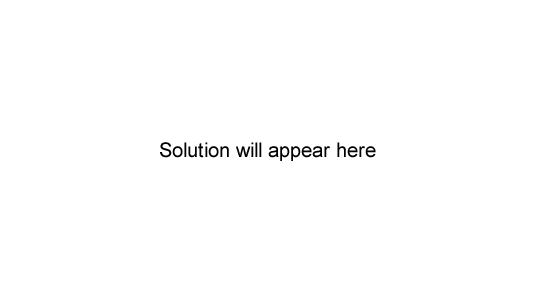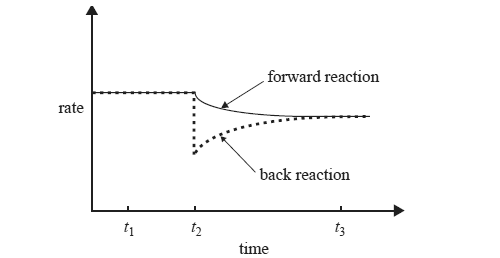Rate of reactions 2009 VCE 1) The addition of a catalyst to a chemical reaction A. lowers the activation energy required for the reaction to occur. B. lowers the chemical energy of the products. C. lowers the chemical energy of the reactants. D. lowers the value of the enthalpy change for the reaction. Solution 2)The two statements below give possible explanations for changes that occur when the temperature of a reaction mixture is increased. I At a higher temperature, particles move faster and the reactant particles collide more frequently. II At a higher temperature, more particles have energy greater than the activation energy. Which alternative below best explains why the observed reaction rate is greater at higher temperatures? A. I only B. II only C. I and II to an equal extent D. I and II, but II to a greater extent than I Solution3) While keeping the temperature constant, the chemist makes a change to a solution of ammonium ions in water that is initially at equilibrium according to the equation below. NH4+(aq) + H2O(l) => H3O+(aq) + NH3(aq) The following graph shows the effect of this change, which was made at time t2, on the rates of the forward and back reactions.What could have caused the change that occurred at time t2? Explain why the rate of the back reaction is affected by this change. Solution